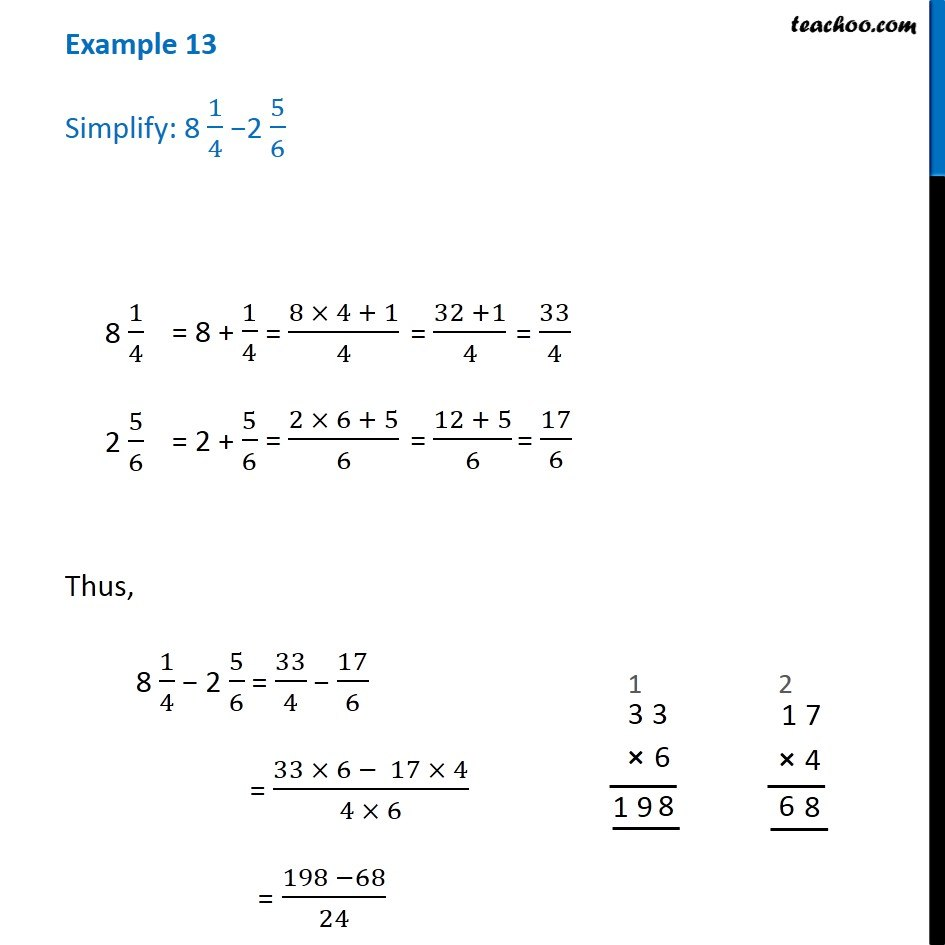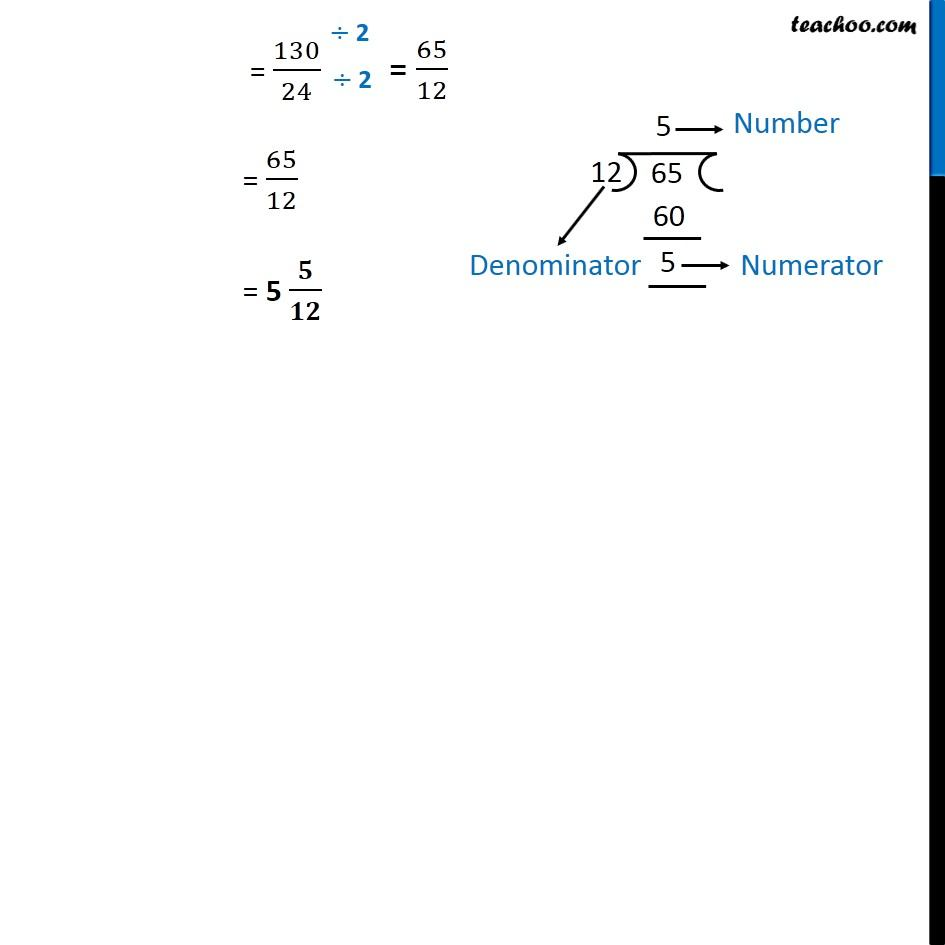Addition and Subtraction of Mixed Fractions

Chapter 7 Class 6 Fractions
Concept wiseIntroducing your new favourite teacher - Teachoo Black, at only ₹83 per month

### Transcript

Example 13 - Chapter 7 Class 6 Fractions (NCERT Books) Simplify: 8 1/4 - 2 5/6 First we simplify the fractions separately 8 1/4 = 8 + 1/4 = (8 x 4 + 1)/4 = (32 + 1)/ 4 = 33/4 2 5/6 = 2 + 5/6 = (2 x 6 + 5)/6 = (12 + 5)/ 6 = 17/6 Thus, 8 1/4 - 2 5/6 = 33/4 - 17/6 = (33 x 6 - 17 x 4) / (4 x 6) = (198 - 68)/24 = 130/24 = 65/12 = 5 𝟓/𝟏𝟐